# Skolem function

(diff) ← Older revision | Latest revision (diff) | Newer revision → (diff)

A concept in predicate logic. If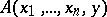is a predicate formula with individual variables (cf. Individual variable), whose domains are sets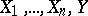, respectively, then a function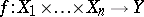is called a Skolem function or resolving function for the formula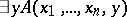if for all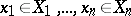one has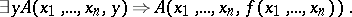Skolem functions were introduced by T. Skolem in the 1920s and have since been widely used in papers on mathematical logic. This is due to the fact that Skolem functions can be used to eliminate alternation of the quantifiers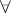and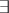. For example, for every formulaof the language of the restricted predicate calculus it is possible to construct a formula of the form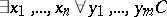(called the Skolem normal form of), wheredoes not contain new quantifiers but does contain new function symbols, such thatis deducible in predicate calculus if and only if its Skolem normal form is.

Skolem functions are used in such fundamental theorems of mathematical logic as Herbrand's theorem, which reduces the question of deducibility of a predicate formula in predicate calculus to that of deducing an infinite sequence of propositional formulas in propositional calculus. They also figure in the Löwenheim–Skolem theorem (cf. Gödel completeness theorem) and elsewhere.

When one deals with formulas on an object domain with an additional structure, one may require a Skolem function to have a definite connection with this structure. For example, if the object domain belongs to the hierarchy of Gödel constructible sets (cf. Gödel constructive set), then one may require that the Skolem functions also belong to a definite level in this hierarchy. It is not always guaranteed that Skolem functions satisfying additional properties exist, but when they do, the effect of using them turns out to be very significant.

By way of an example one can point to Jensen's result on the deducibility of Chang's two-cardinals conjecture (see ) and the negation of the Suslin hypothesis (see ) from Gödel's axiom of constructibility. The Novikov–Kondo theorem on the uniformization of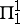-relations from descriptive set theory confirms the existence of a certain type of Skolem function (see ).

How to Cite This Entry:
Skolem function. Encyclopedia of Mathematics. URL: http://encyclopediaofmath.org/index.php?title=Skolem_function&oldid=12101
This article was adapted from an original article by V.N. Grishin (originator), which appeared in Encyclopedia of Mathematics - ISBN 1402006098. See original article